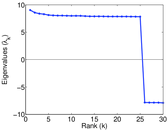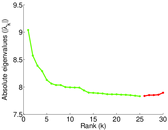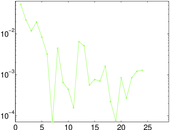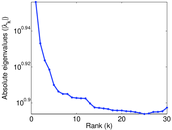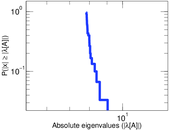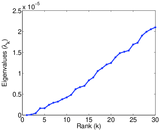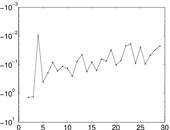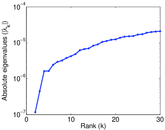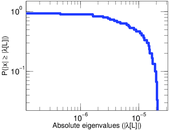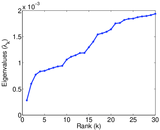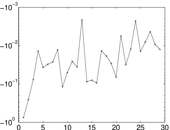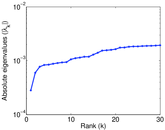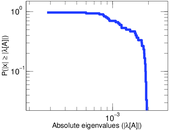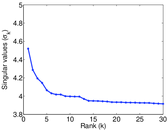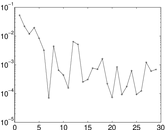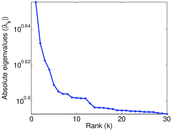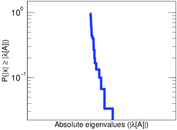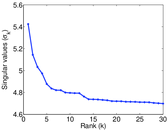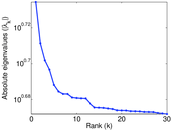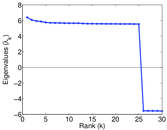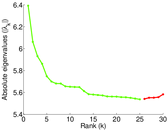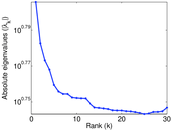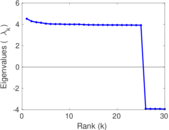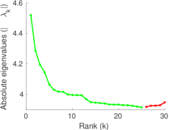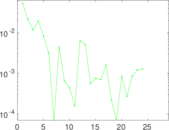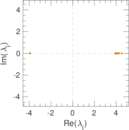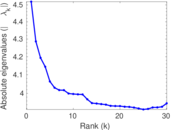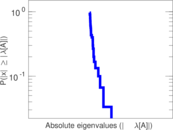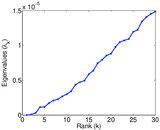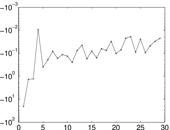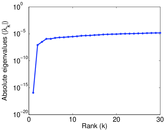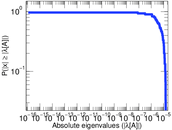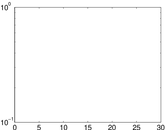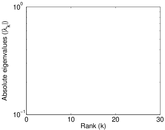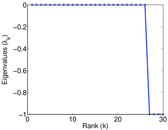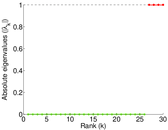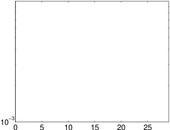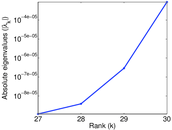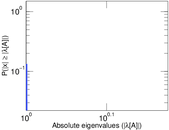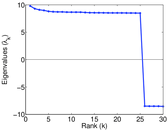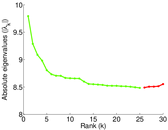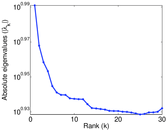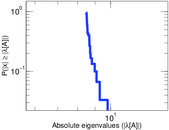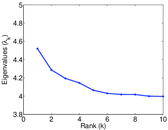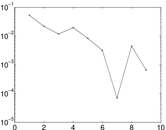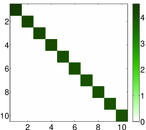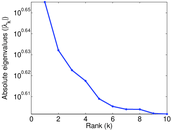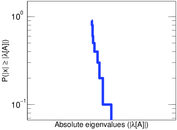# Great Lakes

This is the directed road network from the 9th DIMACS Implementation Challenge, for the area "Great Lakes".

 Code `9L` Internal name `dimacs9-LKS` Name Great Lakes Data source http://www.diag.uniroma1.it/challenge9/download.shtml AvailabilityDataset is available for download Consistency checkDataset passed all tests Category Infrastructure network Dataset timestamp 1991 Node meaning Node Edge meaning Road Network format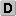Unipartite, directed Edge typeUnweighted, no multiple edges ReciprocalContains reciprocal edges Directed cyclesContains directed cycles LoopsDoes not contain loops

## Statistics

 Size n = 2,758,119 Volume m = 6,794,808 Loop count l = 0 Wedge count s = 6,157,714 Claw count z = 44,551,232 Cross count x = 42,027,094 Triangle count t = 47,051 Square count q = 224,038 4-Tour count T4 = 33,217,968 Maximum degree dmax = 16 Maximum outdegree d+max = 8 Maximum indegree d−max = 8 Average degree d = 4.927 13 Fill p = 8.932 05 × 10−7 Size of LCC N = 2,758,119 Size of LSCC Ns = 2,758,119 Relative size of LSCC Nrs = 1.000 00 Diameter δ = 4,127 50-Percentile effective diameter δ0.5 = 1,104.33 90-Percentile effective diameter δ0.9 = 2,377.70 Median distance δM = 1,105 Mean distance δm = 1,239.73 Gini coefficient G = 0.205 838 Balanced inequality ratio P = 0.423 419 Outdegree balanced inequality ratio P+ = 0.423 419 Indegree balanced inequality ratio P− = 0.423 419 Relative edge distribution entropy Her = 0.994 915 Power law exponent γ = 2.225 85 Tail power law exponent γt = 6.141 00 Tail power law exponent with p γ3 = 6.141 00 p-value p = 0.000 00 Outdegree tail power law exponent with p γ3,o = 6.141 00 Outdegree p-value po = 0.000 00 Indegree tail power law exponent with p γ3,i = 6.141 00 Indegree p-value pi = 0.000 00 Degree assortativity ρ = +0.096 074 9 Degree assortativity p-value pρ = 0.000 00 In/outdegree correlation ρ± = +1.000 00 Clustering coefficient c = 0.022 923 0 Directed clustering coefficient c± = 0.022 923 0 Spectral norm α = 9.039 66 Operator 2-norm ν = 4.519 83 Cyclic eigenvalue π = 4.519 83 Algebraic connectivity a = 1.167 59 × 10−7 Spectral separation |λ1[A] / λ2[A]| = 1.054 40 Reciprocity y = 1.000 00 Non-bipartivity bA = 0.126 633 Normalized non-bipartivity bN = 7.028 07 × 10−5 Algebraic non-bipartivity χ = 0.000 141 024 Spectral bipartite frustration bK = 1.431 10 × 10−5 Controllability C = 272,331 Relative controllability Cr = 0.098 737 9

## Plots

### Degree distribution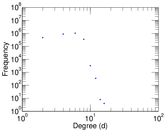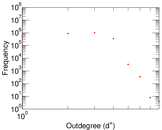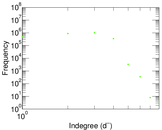### Cumulative degree distribution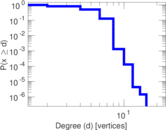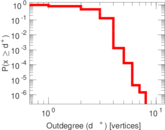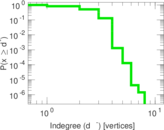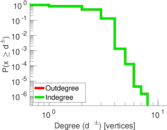### Lorenz curve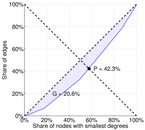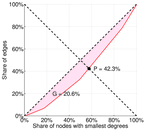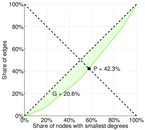### Spectral distribution of the adjacency matrix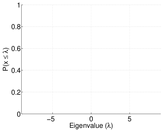### Spectral distribution of the normalized adjacency matrix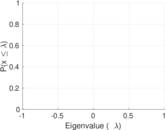### Spectral distribution of the Laplacian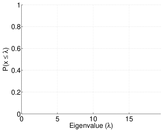### Spectral graph drawing based on the adjacency matrix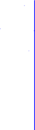### Spectral graph drawing based on the Laplacian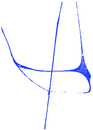### Spectral graph drawing based on the normalized adjacency matrix### Hop distribution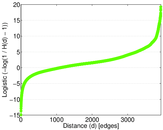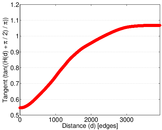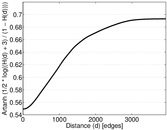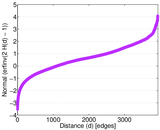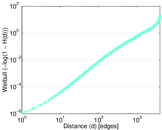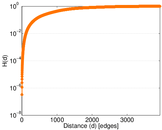### In/outdegree scatter plot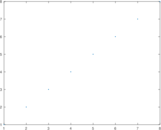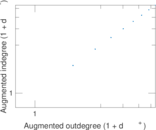### SynGraphy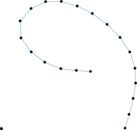### Matrix decompositions plots